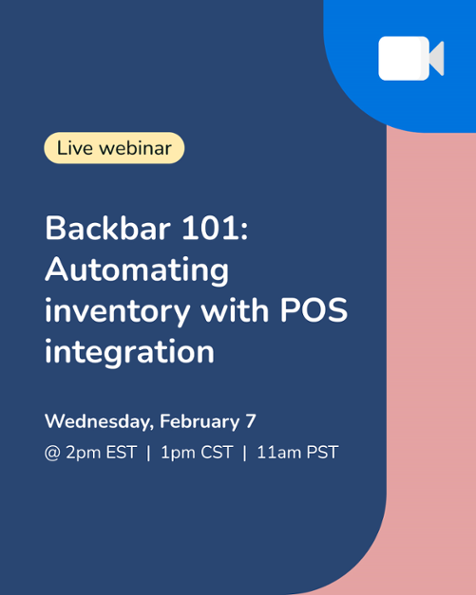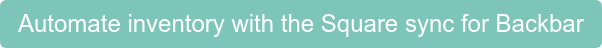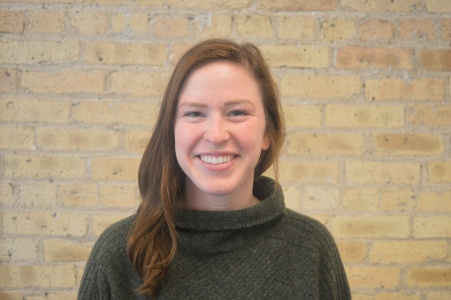There are many costs that go into running a restaurant and while some are not controllable, others are, and taking advantage of lowering those costs will lead to a successful business.  The costs that are easiest to control in a restaurant include, or what some call "The Big Three":

• Liquor Costs
• Food Costs
• Labor Costs

We will go over each in more detail and the industry averages and show you how to calculate them so you can see how your costs are impacting your business.

## Prime Costs

The sum of your Cost of Goods Sold (COGS) for food and beverage and labor costs is called your prime costs.  These costs include food and beverage inventory, labor, payroll taxes, any workers' compensation, medical insurance, and employee benefits.  Your prime costs will give you an indication of how much you should charge in order to say profitable and how much you can spend without running yourself into debt.

Total COGS + Total Labor = Prime Costs

Just in case you forgot how to calculate COGS here is the formula:

Beginning Inventory + Purchases - Ending Inventory = COGS

Beginning Inventory = the amount of food and beverage you started with on your shelves for a certain period.

Purchases = the amount of food and beverage you bought for a certain period.

Ending Inventory = the amount of food and beverage left over for a certain period.

#### Prime Costs as a Percentage of Sales

You will also want to compare your prime costs to your total sales to see if you are within a healthy range.

Prime Cost as Percentage of Sales = Prime Cost/Total Sales

Ideally you would want your prime costs to be between 55-65% and the rest goes to other expenses like rent, new equipment, utilities and menu design.  Anything less than 50% can indicate your food quality is low, you are charging too much, or have too little staff.  On the other hand, anything over 70% makes it hard to be profitable because you only have 30% to go to your other expenses such as rent, insurance and utilities.

For example, say your labor costs were \$4,000 for a given period and your food and beverage COGS were \$8,000.  Your prime costs would be \$12,000.

(4,000 + 8,000) = \$12,000.

Now say your total sales for the period were \$19,000.  Your prime cost as a percentage would be 63%

(12,000 / 19,000 x 100) = .63 or 63%.

63% is in the ideal range for a prime cost percentage and this means you have 37% that can be allocated for rent, utilities, insurance and other expenses and also profits.

Now that we've gone over how much your prime costs should ideally be, we can dive in and see what the typical costs are for liquor, food, and labor for a restaurant or bar.

## Average Liquor Costs

Obviously every bar and restaurant would love to keep their liquor costs as low as possible, because the lower the liquor costs, the greater the profits.  Your liquor costs are based on your direct costs and gross margin and every place has different costs to take into account that will affect liquor costs.

The industry average for total beverage programs is between 18-24%.  Every drink type has a different cost percentage as well.  Liquor is about 15%, draft beer about 20%, bottled beer around 25%, and wine can be upwards of 30-40%.#### How to Calculate Your Liquor Costs

To calculate your liquor costs, you want to take your Cost of Goods Sold and divide by total sales.

COGS/Liquor Sales = Liquor Costs

For example, if for the year your bar sold \$15,000 of liquor inventory and it generated sales of \$65,000 then your liquor cost percentage would be 23%.  So every dollar in sales means 23 cents is used to pay for the liquor and the other 77 cents is your gross margin.  Remember that gross margin is the amount of sales revenue after taking out the costs to produce the item.

(15,000/65,000) = .23 x 100 = 23%

## Average Food Costs

Because of slim margins, controlling food costs is one of the biggest challenges for restaurant operators.  By monitoring these costs closely you will know when to adjust prices, buy different products or quantities, or change distributors.  Typically the average food costs are around 28-35% to remain profitable.

#### How to Calculate Food Costs

Your COGS for food is the amount you spend on ingredients for each dish and inventory for a given time period.  To calculate actual food costs use the following formula:

Food Cost Percentage = Beginning Inventory + Purchases - Ending Inventory / Food Sales

For example, let's say you had \$8,000 in beginning inventory, purchases of \$1,500 and an ending inventory of \$7,500 and \$6,000 in sales for a given period.  You would have a food cost of 33% so for every dollar in sales it costs you 33 cents.

(\$8,000 + 1,500) - 7,500 / 6,000 = .33 x 100 = 33%

#### How to Calculate Ideal Food Costs

Anyone can add up food costs, but without comparing it to something what does it even mean?  Looking at your ideal food costs can show you any inconsistencies between your food cost percentage and your actual food cost.  Unlike actual food costs, ideal food costs do not consider beginning and ending inventories, but looks at total costs and sales. The formula for ideal food costs is:

Ideal Food Cost Percentage = Total Cost Per Dish / Total Sales Per Dish

For example, say your total cost per dish is \$1,500 and total sales per dish is \$6,000. Your ideal food cost percentage would be 25%.

(\$1,500 / 6,000) = .25 or 25%

So looking at the ideal food cost percentage and the actual food cost percentage we can see there is a 8% difference (33% - 25%).  This percentage difference can come from food waste or over ordering, which now that we know this we can take steps to reduce costs.

## Average Labor Costs

First of all, what is labor cost?  You might be thinking it is just your hourly wages and salaried employees, but there is more to it.  Labor costs are anything that is labor-related and include:

• Salaried employees
• Hourly employees
• Bonuses
• Overtime
• Payroll taxes
• Health care
• Vacation and sick days

A good rule of thumb is to aim to keep labor costs between 20-30% of gross revenue.  With that being said, every establishment is different and sometimes you require more staff on hand than usual that might increase your costs and other times you are able to cut staff to reduce labor costs.  A fast-food restaurant could typically run labor costs around 25% while a full service restaurant could run about 30-40% of revenue depending on how up scale the bar or restaurant is and the demand needed.#### How to Calculate Labor Costs

In order to determine what your restaurant is running at for labor costs you will need to calculate labor costs as a percentage.  The first thing you will want to do is add up the cost from the different cost groups.  For example let's say your labor costs are as follows for a given year:

• Salaries and wages: 140,000+
• Bonuses: 12,000+
• Overtime: 28,000+
• Payroll taxes: 20,000+
• Health care: 30,000+
• Vacation and sick days: 7,000+
• Total labor costs: 237,000

#### How to Calculate Labor Costs as a Percentage

Looking at the total labor costs doesn't tell us much until we convert it to a percentage of sales.  There are two ways to go about this.  This first is by calculating labor costs as a percentage of total sales and the other as a percentage of operating costs.

Total Labor Costs/Total Sales

First you will want to collect your total revenue data from your income statement or POS system report.  For example let's assume your sales for the year were \$900,000.

Next, you will want to calculate your total labor costs by adding up all the categories.  We will use the number from above of \$237,000.

You will then use the formula and divide labor cost by revenue.  Your labor costs would be 26% of your sales, which is right within the industry average.

\$237,000 / 900,000 x 100 = .26 or 26%

The second way to calculate labor costs is as a percentage of operating costs.  You would use the same process as above, but rather than using revenue you would use operating costs.

Total Labor Costs/Total Operating Costs

Track your food/beverage and labor cost percentages over time and frequently to make sure you are staying within reach of your goals.  If you see variances or increases in your percentage you will want to take additional steps to control and lower those costs like finding a different distributor or cutting staff when necessary.